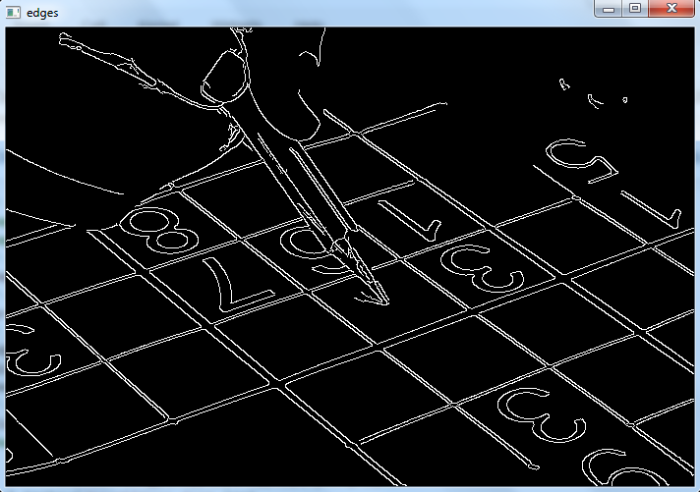# How to implement probabilistic Hough Transform in OpenCV Python?

Probabilistic Hough Transform is an optimization of the Hough Transform. The hough transform takes a lot of computation even for a line with two arguments. Probabilistic Hough Transform doesn't take all the points into consideration, it takes only a random subset of points and that is sufficient for line detection. We could follow the below given steps to implement probabilistic Hough Transform on an image-

• Import the required library. In all the following examples, the required Python library is OpenCV. Make sure you have already installed it.

• Read an input image using cv2.imread(). The RGB image read using this method is in BGR format. Optionally assign the read BGR image to img.

• Now convert this BGR image to grayscale image as below using cv2.cvtColor() function. Optionally assign the converted grayscale image to gray.

• Apply thresholding on the grayscale image to convert it to a binary image. Adjust the second parameter (threshValue) for better binary image.

• Find the edges in the binary image using Canny edge detector (you can use any other edge detector to detect edges).

edges = cv2.Canny(gray,50,200,apertureSize = 3)

• Apply probabilistic Hough transform on the edge image using cv2.HoughLinesP(). It returns the coordinates of detected lines.

lines = cv2.HoughLinesP(edges, 1, np.pi/180, 50, minLineLength, maxLineGap)

• Draw lines on the image and display the output image.

Let's have a look at some examples for more clear understanding.

## Input Image

We will use this image as an input file in the examples below.## Example

In this example, we find the probabilistic Hough Line transform on the image with the following line properties −

• minLineLength = 10

• maxLineGap = 5

# import required libraries
import cv2
import numpy as np

# convert the input image to grayscale image
gray = cv2.cvtColor(img,cv2.COLOR_BGR2GRAY)

# find the edges using Canny edge detector
edges = cv2.Canny(gray,50,200,apertureSize = 3)
minLineLength = 10
maxLineGap = 5

# apply probabilistic Hough transform
lines = cv2.HoughLinesP(edges,1,np.pi/180,50,minLineLength,maxLineGap)
for line in lines:
for x1,y1,x2,y2 in line:
cv2.line(img,(x1,y1),(x2,y2),(0,255,0),2)
cv2.imshow('houghlines.jpg',img)
cv2.imshow('edges', edges)
cv2.waitKey(0)
cv2.destroyAllWindows()


## Output

When you run the above program, it will produce the following windows −The first window "Edges" shows the edges detected using Canny edge detection algorithm. The second window "houghlines.jpg" shows the detected lines using probabilistic Hough Line transform.

Updated on: 02-Dec-2022

2K+ Views